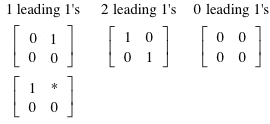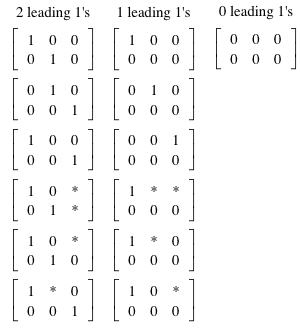# Listing Possible RREF Matrix Combinations

MDS

## Homework Statement

Using the values 1, 0, and *(to represent any number), list all the possible combinations that could result from performing reduced row echelon form on a 2 x 3 matrix.

## Homework Equations

Instructor 2 x 2 matrix example:## The Attempt at a Solution

I have found 13 different combinations:but we have no examples beyond the 2 x 2 matrix so I haven't been able to attempt to model an equation(since I can't find a theorem or definition that models this) to predict the number of combinations I should find for a 2 x 3 matrix.

Any help in determining if this is the correct amount of combinations for a 2 x 3 matrix that has been simplified to RREF or what ones I may be missing would be appreciated.

Aryan patel
how to find all possible rref matrix for m*n matrix. how I create a formula for m*n matrix

Homework Helper
Gold Member
how to find all possible rref matrix for m*n matrix. how I create a formula for m*n matrix
Start by listing all the constraints that apply to such a matrix.

By the way, I believe @MDS was overcounting in the 2x3 case. You should not count separately a case which can be got by replacing some * with 0s and 1s from another case.
E.g
1**
000
subsumes
100
000

For 2x3, the general formula I get gives 7.

Last edited:
Dhruv shrivastava